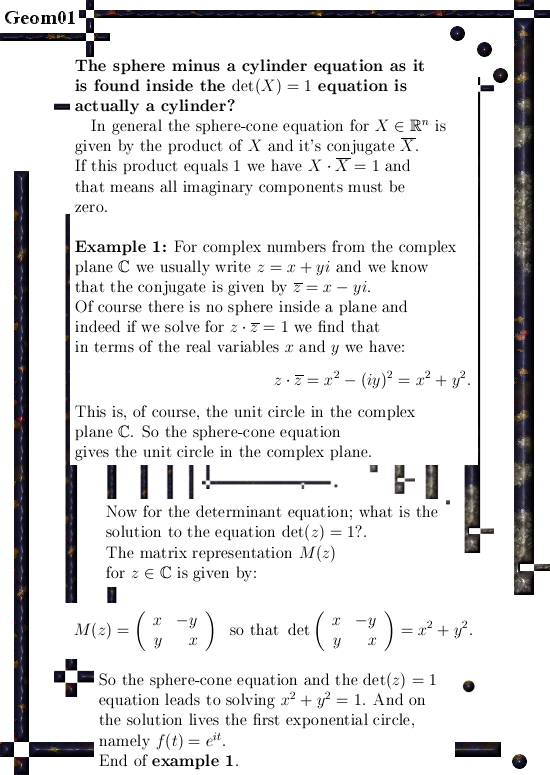# On a way to find more equations so that the 1D existence of exponential curves in all possible dimensions is assured.

In part this post picks up where I left the stuff of the missing equations back in the year 2015. The missing equations are found inside the determinant equation; for this to succeed we must factorize determinant of the matrix representations of higher dimensional numbers. A well known result from linear algebra is that the determinant is also the product of the eigen values; so we need to craft the eigen value functions that for every X in our higher dimensional number space give the eigen values.

These eigenvalue functions are also the discrete Fourier transform of our beloved higher dimensional numbers and these functions come in conjugate pairs. Such a pair form two factors of the determinant and if we multiply them we can get rid of all complex coefficients from the complex plane.

A rather surprising result is the fact that if we subtract a cone equation from a sphere equation we get a cylinder…

This post is also a way of viewing the exponential circles and curves as an intersection of all kinds of geometric objects like the unit sphere, (hyper) cones, (hyper) planes and (hyper cylinders. Usually I represent it all as some analysis but you can take a very geometric approach too.

I have no idea if the shape of the higher dimensional curves is studied as a geometrical object; I suspect this is not the case since the use of complex numbers outside the complex plane is very seldom observed. The professionals just want their tiny fishing bowl (the complex plane) and declare it an Olympic swimming pool…
Well, let it be because these people will never change.

All in all this post is 20 pictures long (size 550 x 775) so it is a relatively long read.The pictures of the graphs were all made with an applet named Animated drawing, here is a link and there you can find it under ´Online calculators and function plotters´±

For example you can cut and paste the next five dimensional equations that represents a hypercone going through all the coordinate axis:

((1/5)*sin(5*x)/sin(x))*((1/5)*sin(5*(x-2*pi/5))/sin(x-2*pi/5)) +
((1/5)*sin(5*(x-2*pi/5))/sin(x-2*pi/5))*((1/5)*sin(5*(x-4*pi/5))/sin(x-4*pi/5)) +
((1/5)*sin(5*(x-4*pi/5))/sin(x-4*pi/5))*((1/5)*sin(5*(x-pi/5))/sin(x-pi/5)) +
((1/5)*sin(5*(x-pi/5))/sin(x-pi/5))*((1/5)*sin(5*(x-3*pi/5))/sin(x-3*pi/5)) +
((1/5)*sin(5*(x-3*pi/5))/sin(x-3*pi/5))*((1/5)*sin(5*x)/sin(x))

The above thing should give identical zero for all x.
An important feature of exponential curves in spaces with an odd number of dimensions is that they all are inside a hyperplane. The hyperplane says the sum of the coordinates is always 1. If you cut and past the next sum of the five coordinate functions you see that you always get one for all x:

((1/5)*sin(5*x)/sin(x)) +
((1/5)*sin(5*(x-pi/5))/sin(x-pi/5)) +
((1/5)*sin(5*(x-2*pi/5))/sin(x-2*pi/5)) +
((1/5)*sin(5*(x-3*pi/5))/sin(x-3*pi/5)) +
((1/5)*sin(5*(x-4*pi/5))/sin(x-4*pi/5))

At last the link to the original update from 2015 where I found the missing equations for the first time. But all I knew they were hidden inside the determinant. A few weeks ago I decided to take a better look and the result is this post.

From 14 July 2015: The missing equations.
http://kinkytshirts.nl/rootdirectory/just_some_math/3d_complex_stuff03.htm#14July2015

# On an old idea that did not work…

Recently I am working on a relatively long post where I try to take a much more geometric approach to finding exponential circles and exponential curves. That post is also going forward from a few years back when I was searching for the so called missing equations.

The problem of the missing equations does not arise until you start working in five dimensions or higher; the equations as generated by the sphere-cone equations are just not enough to end up with a one dimensional curve.
Back in the time I simply took a few weeks until I had found the answer: The missing equations can be found inside the determinant!

For example if you have a 17 by 17 matrix in 17 variables (the so called matrix representations of 17-dimensional number systems), all you have to do is factorize this determinant and from those factors you can craft the extra needed equations.

Weirdly enough you find a hyper plane and a bunch of hyper-cylinders.

So in the next post I try to show you how you can have a very geometric approach to finding the higher dimensional exponential curves as the intersection of a sphere, a hyperplane, a bunch of cones and a bunch of cylinders.

__________

In my old notes I found a mysterious looking line of squares of cosines with their time lags. That was from before I solved the problem and this ‘solution’ has all kinds of faults in it.

That is this small post; it is about something that does not work.
It is just 3 pictures long (550 x 775 pixels):

It was just over two years back I wrote that long update on the other website about the missing equations, I was glad I took those weeks to solve this problem because it is crucial for the development of general higher dimensional theory on this detail.

Here is a link to that old update±

I hope next week I am ready with the new long post, after that I will likely pick up magnetism again because I finally found out what the professionals mean when talking about ´inverted V-s’; that means there is also an electric field accelerating the particles in the aurora’s of earth and Jupiter.

Tiny problem for the professionals: At the Jupiter site, regardless of inverted V-s yes or no, the plasma particles get accelerated anyway…
So that looks like one more victory for Reinko Venema and one more silence from the professional professors.

See ya around!